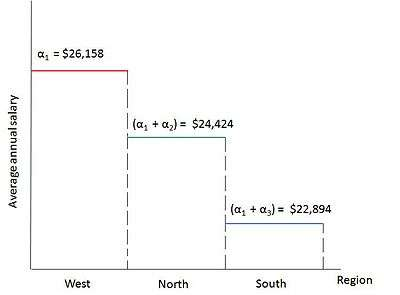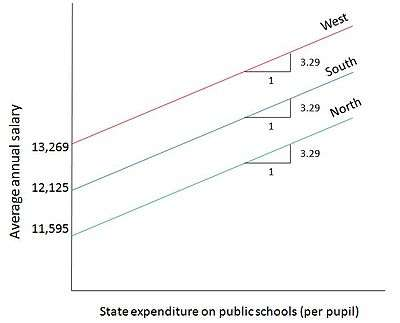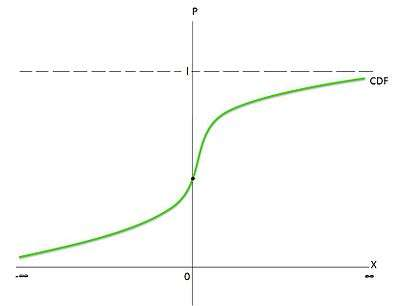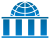# Dummy variable (statistics)

In statistics and econometrics, particularly in regression analysis, a dummy variable (also known as an indicator variable, design variable, Boolean indicator, categorical variable, binary variable, or qualitative variable) is one that takes the value 0 or 1 to indicate the absence or presence of some categorical effect that may be expected to shift the outcome. Dummy variables are used as devices to sort data into mutually exclusive categories (such as smoker/non-smoker, etc.). For example, in econometric time series analysis, dummy variables may be used to indicate the occurrence of wars or major strikes. A dummy variable can thus be thought of as a truth value represented as a numerical value 0 or 1 (as is sometimes done in computer programming).

Dummy variables are "proxy" variables or numeric stand-ins for qualitative facts in a regression model. In regression analysis, the dependent variables may be influenced not only by quantitative variables (income, output, prices, etc.), but also by qualitative variables (gender, religion, geographic region, etc.). A dummy independent variable (also called a dummy explanatory variable) which for some observation has a value of 0 will cause that variable's coefficient to have no role in influencing the dependent variable, while when the dummy takes on a value 1 its coefficient acts to alter the intercept. For example, suppose Gender is one of the qualitative variables relevant to a regression. Then, female and male would be the categories included under the Gender variable. If female is arbitrarily assigned the value of 1, then male would get the value 0. Then the intercept (the value of the dependent variable if all other explanatory variables hypothetically took on the value zero) would be the constant term for males but would be the constant term plus the coefficient of the gender dummy in the case of females.

Dummy variables are used frequently in time series analysis with regime switching, seasonal analysis and qualitative data applications. Dummy variables are involved in studies for economic forecasting, bio-medical studies, credit scoring, response modelling, etc. Dummy variables may be incorporated in traditional regression methods or newly developed modeling paradigms.

## Incorporating a dummy independentFigure 1 : Graph showing wage = α0 + δ0female + α1education + U, δ0 < 0.

Dummy variables are incorporated in the same way as quantitative variables are included (as explanatory variables) in regression models. For example, if we consider a Mincer-type regression model of wage determination, wherein wages are dependent on gender (qualitative) and years of education (quantitative):whereis the error term. In the model, female = 1 when the person is a female and female = 0 when the person is male.can be interpreted as: the difference in wages between females and males, holding education constant. Thus, δ0 helps to determine whether there is a discrimination in wages between males and females. For example, if δ0>0 (positive coefficient), then women earn a higher wage than men (keeping other factors constant). Note that the coefficients attached to the dummy variables are called differential intercept coefficients. The model can be depicted graphically as an intercept shift between females and males. In the figure, the case δ0<0 is shown (wherein, men earn a higher wage than women).

Dummy variables may be extended to more complex cases. For example, seasonal effects may be captured by creating dummy variables for each of the seasons:if the observation is for summer, and equals zero otherwise;if and only if autumn, otherwise equals zero;if and only if winter, otherwise equals zero; andif and only if spring, otherwise equals zero. In the panel data fixed effects estimator dummies are created for each of the units in cross-sectional data (e.g. firms or countries) or periods in a pooled time-series. However in such regressions either the constant term has to be removed or one of the dummies has to be removed, with its associated category becoming the base category against which the others are assessed in order to avoid the dummy variable trap:

The constant term in all regression equations is a coefficient multiplied by a regressor equal to one. When the regression is expressed as a matrix equation, the matrix of regressors then consists of a column of ones (the constant term), vectors of zeros and ones (the dummies), and possibly other regressors. If one includes both male and female dummies, say, the sum of these vectors is a vector of ones, since every observation is categorized as either male or female. This sum is thus equal to the constant term's regressor, the first vector of ones. As result, the regression equation will be unsolvable, even by the typical pseudoinverse method. In other words: if both the vector-of-ones (constant term) regressor and an exhaustive set of dummies are present, perfect multicollinearity occurs, and the system of equations formed by the regression does not have a unique solution. This is referred to as the dummy variable trap. The trap can be avoided by removing either the constant term or one of the offending dummies. The removed dummy then becomes the base category against which the other categories are compared.

## ANOVA models

Main article: Analysis of variance

A regression model in which the dependent variable is quantitative in nature but all the explanatory variables are dummies (qualitative in nature) is called an Analysis of Variance (ANOVA) model.

### ANOVA model with one qualitative variable

Suppose we want to run a regression to find out if the average annual salary of public school teachers differs among three geographical regions in Country A with 51 states: (1) North (21 states) (2) South (17 states) (3) West (13 states). Say that the simple arithmetic average salaries are as follows: $24,424.14 (North),$22,894 (South), $26,158.62 (West). The arithmetic averages are different, but are they statistically different from each other? To compare the mean values, Analysis of Variance techniques can be used. The regression model can be defined as:, whereaverage annual salary of public school teachers in state iif the state i is in the North Regionotherwise (any region other than North)if the state i is in the South Regionotherwise In this model, we have only qualitative regressors, taking the value of 1 if the observation belongs to a specific category and 0 if it belongs to any other category. This makes it an ANOVA model.Figure 2 : Graph showing the regression results of the ANOVA model example: Average annual salaries of public school teachers in 3 regions of Country A. Now, taking the expectation of both sides, we obtain the following: Mean salary of public school teachers in the North Region: E(Yi|D2i = 1, D3i = 0) = α1 + α2 Mean salary of public school teachers in the South Region: E(Yi|D2i = 0, D3i = 1) = α1 + α3 Mean salary of public school teachers in the West Region: E(Yi|D2i = 0, D3i = 0) = α1 (The error term does not get included in the expectation values as it is assumed that it satisfies the usual OLS conditions, i.e., E(Ui) = 0) The expected values can be interpreted as follows: The mean salary of public school teachers in the West is equal to the intercept term α1 in the multiple regression equation and the differential intercept coefficients, α2 and α3, explain by how much the mean salaries of teachers in the North and South Regions vary from that of the teachers in the West. Thus, the mean salaries of teachers in the North and South is compared against the mean salary of the teachers in the West. Hence, the West Region becomes the base group or the benchmark group,i.e., the group against which the comparisons are made. The omitted category, i.e., the category to which no dummy is assigned, is taken as the base group category. Using the given data, the result of the regression would be: Ŷi = 26,158.62 − 1734.473D2i − 3264.615D3i se = (1128.523) (1435.953) (1499.615) t = (23.1759) (−1.2078) (−2.1776) p = (0.0000) (0.2330) (0.0349) R2 = 0.0901 where, se = standard error, t = t-statistics, p = p value The regression result can be interpreted as: The mean salary of the teachers in the West (base group) is about$26,158, the salary of the teachers in the North is lower by about $1734 ($26,158.62 − $1734.473 =$24.424.14, which is the average salary of the teachers in the North) and that of the teachers in the South is lower by about $3265 ($26,158.62 − $3264.615 =$22,894, which is the average salary of the teachers in the South).

To find out if the mean salaries of the teachers in the North and South are statistically different from that of the teachers in the West (the comparison category), we have to find out if the slope coefficients of the regression result are statistically significant. For this, we need to consider the p values. The estimated slope coefficient for the North is not statistically significant as its p value is 23 percent; however, that of the South is statistically significant at the 5% level as its p value is only around 3.5 percent. Thus the overall result is that the mean salaries of the teachers in the West and North are not statistically different from each other, but the mean salary of the teachers in the South is statistically lower than that in the West by around $3265. The model is diagrammatically shown in Figure 2. This model is an ANOVA model with one qualitative variable having 3 categories. ### ANOVA model with two qualitative variables Suppose we consider an ANOVA model having two qualitative variables, each with two categories: Hourly Wages are to be explained in terms of the qualitative variables Marital Status (Married / Unmarried) and Geographical Region (North / Non-North). Here, Marital Status and Geographical Region are the two explanatory dummy variables. Say the regression output on the basis of some given data appears as follows: Ŷi = 8.8148 + 1.0997D2 − 1.6729D3 where, Y = hourly wages (in$)
D2 = marital status, 1 = married, 0 = otherwise
D3 = geographical region, 1 = North, 0 = otherwise

In this model, a single dummy is assigned to each qualitative variable, one less than the number of categories included in each.

Here, the base group is the omitted category: Unmarried, Non-North region (Unmarried people who do not live in the North region). All comparisons would be made in relation to this base group or omitted category. The mean hourly wage in the base category is about $8.81 (intercept term). In comparison, the mean hourly wage of those who are married is higher by about$1.10 and is equal to about $9.91 ($8.81 + $1.10). In contrast, the mean hourly wage of those who live in the North is lower by about$1.67 and is about $7.14 ($8.81 − $1.67). Thus, if more than one qualitative variable is included in the regression, it is important to note that the omitted category should be chosen as the benchmark category and all comparisons will be made in relation to that category. The intercept term will show the expectation of the benchmark category and the slope coefficients will show by how much the other categories differ from the benchmark (omitted) category. ## ANCOVA models A regression model that contains a mixture of both quantitative and qualitative variables is called an Analysis of Covariance (ANCOVA) model. ANCOVA models are extensions of ANOVA models. They statistically control for the effects of quantitative explanatory variables (also called covariates or control variables). To illustrate how qualitative and quantitative regressors are included to form ANCOVA models, suppose we consider the same example used in the ANOVA model with one qualitative variable: average annual salary of public school teachers in three geographical regions of Country A. If we include a quantitative variable, State Government expenditure on public schools per pupil, in this regression, we get the following model:Figure 3 : Graph showing the regression results of the ANCOVA model example: Public school teacher's salary (Y) in relation to State expenditure per pupil on public schools. Yi = α1 + α2D2i + α3D3i + α4Xi + Ui where, Yi = average annual salary of public school teachers in state i Xi = State expenditure on public schools per pupil D2i = 1, if the State i is in the North Region D2i = 0, otherwise D3i = 1, if the State i is in the South Region D3i = 0, otherwise Say the regression output for this model is Ŷi = 13,269.11 1673.514D2i 1144.157D3i + 3.2889Xi The result suggests that, for every$1 increase in State expenditure per pupil on public schools, a public school teacher's average salary goes up by about $3.29. Further, for a state in the North region, the mean salary of the teachers is lower than that of West region by about$1673 and for a state in the South region, the mean salary of teachers is lower than that of the West region by about $1144. Figure 3 depicts this model diagrammatically. The average salary lines are parallel to each other by the assumption of the model that the coefficient of expenditure does not vary by state. The trade off shown separately in the graph for each category is between the two quantitative variables: public school teachers' salaries (Y) in relation to State expenditure per pupil on public schools (X). ## Interactions among dummy variables Quantitative regressors in regression models often have an interaction among each other. In the same way, qualitative regressors, or dummies, can also have interaction effects between each other, and these interactions can be depicted in the regression model. For example, in a regression involving determination of wages, if two qualitative variables are considered, namely, gender and marital status, there could be an interaction between marital status and gender. These interactions can be shown in the regression equation as illustrated by the example below. With the two qualitative variables being gender and marital status and with the quantitative explanator being years of education, a regression that is purely linear in the explanators would be Yi = β1 + β2D2,i + β3D3,i + αXi + Ui where i denotes the particular individual Y = Hourly Wages (in$)
X = Years of education
D2 = 1 if female, 0 otherwise
D3 = 1 if married, 0 otherwise

This specification does not allow for the possibility that there may be an interaction that occurs between the two qualitative variables, D2 and D3. For example, a female who is married may earn wages that differ from those of an unmarried male by an amount that is not the same as the sum of the differentials for solely being female and solely being married. Then the effect of the interacting dummies on the mean of Y is not simply additive as in the case of the above specification, but multiplicative also, and the determination of wages can be specified as:

Yi = β1 + β2D2,i + β3D3,i + β4(D2,iD3,i) + αXi + Ui

Here,

β2 = differential effect of being a female
β3 = differential effect of being married
β4 = further differential effect of being both female and married

By this equation, in the absence of a non-zero error the wage of an unmarried male is β1+ αXi, that of an unmarried female is β1+ β2 + αXi, that of being a married male is β1+ β3 + αXi, and that of being a married female is β12+ β3 + β4+ αXi (where any of the estimates of the coefficients of the dummies could turn out to be positive, zero, or negative).

Thus, an interaction dummy (product of two dummies) can alter the dependent variable from the value that it gets when the two dummies are considered individually.

However, the use of products of dummy variables to capture interactions can be avoided by using a different scheme for categorizing the dataone that specifies categories in terms of combinations of characteristics. If we let

D4 = 1 if unmarried female, 0 otherwise
D5 = 1 if married male, 0 otherwise
D6 = 1 if married female, 0 otherwise

then it suffices to specify the regression

Yi = δ1 + δ4D4,i + δ5D5,i + δ6D6,i + αXi + Ui.

Then with zero shock term the value of the dependent variable is δ1+ αXi for the base category unmarried males, δ1 + δ4+ αXi for unmarried females, δ1 + δ5+ αXi for married males, and δ1 + δ6+ αXi for married females. This specification involves the same number of right-side variables as does the previous specification with an interaction term, and the regression results for the predicted value of the dependent variable contingent on Xi, for any combination of qualitative traits, are identical between this specification and the interaction specification.

## Dummy dependent variables

### What happens if the dependent variable is a dummy?

A model with a dummy dependent variable (also known as a qualitative dependent variable) is one in which the dependent variable, as influenced by the explanatory variables, is qualitative in nature. Some decisions regarding 'how much' of an act must be performed involve a prior decision making on whether to perform the act or not. For example, the amount of output to produce, the cost to be incurred, etc. involve prior decisions on whether to produce or not, whether to spend or not, etc. Such "prior decisions" become dependent dummies in the regression model.

For example, the decision of a worker to be a part of the labour force becomes a dummy dependent variable. The decision is dichotomous, i.e., the decision has two possible outcomes: yes and no. So the dependent dummy variable Participation would take on the value 1 if participating, 0 if not participating. Some other examples of dichotomous dependent dummies are cited below:

Decision: Choice of Occupation. Dependent Dummy: Supervisory = 1 if supervisor, 0 if not supervisor.

Decision: Affiliation to a Political Party. Dependent Dummy: Affiliation = 1 if affiliated to the party, 0 if not affiliated.

Decision: Retirement. Dependent Dummy: Retired = 1 if retired, 0 if not retired.

When the qualitative dependent dummy variable has more than two values (such as affiliation to many political parties), it becomes a multiresponse or a multinomial or polychotomous model.

### Dependent dummy variable models

Analysis of dependent dummy variable models can be done through different methods. One such method is the usual OLS method, which in this context is called the linear probability model. An alternative method is to assume that there is an unobservable continuous latent variable Y* and that the observed dichotomous variable Y = 1 if Y* > 0, 0 otherwise. This is the underlying concept of the logit and probit models. These models are discussed in brief below.

#### Linear probability model

An ordinary least squares model in which the dependent variable Y is a dichotomous dummy, taking the values of 0 and 1, is the linear probability model (LPM). Suppose we consider the following regression:where= family incomeif a house is owned by the family, 0 if a house is not owned by the family

The model is called the linear probability model because, the regression is linear. The conditional mean of Yi given Xi, written as, is interpreted as the conditional probability that the event will occur for that value of Xi — that is, Pr(Yi = 1 |Xi). In this example,gives the probability of a house being owned by a family whose income is given by Xi.

Now, using the OLS assumption, we getSome problems are inherent in the LPM model:

1. The regression line will not be a well-fitted one and hence measures of significance, such as R2, will not be reliable.
2. Models that are analyzed using the LPM approach will have heteroscedastic disturbances.
3. The error term will have a non-normal distribution.
4. The LPM may give predicted values of the dependent variable that are greater than 1 or less than 0. This will be difficult to interpret as the predicted values are intended to be probabilities, which must lie between 0 and 1.
5. There might exist a non-linear relationship between the variables of the LPM model, in which case, the linear regression will not fit the data accurately.

#### Alternatives to LPMFigure 4 : A cumulative distribution function.

To avoid the limitations of the LPM, what is needed is a model that has the feature that as the explanatory variable, Xi, increases, Pi = E (Yi = 1 | Xi) should remain within the range between 0 and 1. Thus the relationship between the independent and dependent variables is necessarily non-linear.

For this purpose, a cumulative distribution function (CDF) can be used to estimate the dependent dummy variable regression. Figure 4 shows an 'S'-shaped curve, which resembles the CDF of a random variable. In this model, the probability is between 0 and 1 and the non-linearity has been captured. The choice of the CDF to be used is now the question.

Two alternative CDFs can be used: the logistic and normal CDFs. The logistic CDF gives rise to the logit model and the normal CDF give rises to the probit model .

#### Logit model

Main article: Logistic regression

The shortcomings of the LPM led to the development of a more refined and improved model called the logit model. In the logit model, the cumulative distribution of the error term in the regression equation is logistic. The regression is more realistic in that it is non-linear.

The logit model is estimated using the maximum likelihood approach. In this model,, which is the probability of the dependent variable taking the value of 1 given the independent variable is:where.

The model is then expressed in the form of the odds ratio: what is modeled in the logistic regression is the natural logarithm of the odds, the odds being defined as. Taking the natural log of the odds, the logit (Li) is expressed asThis relationship shows that Li is linear in relation to Xi, but the probabilities are not linear in terms of Xi.

#### Probit model

Main article: Probit model

Another model that was developed to offset the disadvantages of the LPM is the probit model. The probit model uses the same approach to non-linearity as does the logit model; however, it uses the normal CDF instead of the logistic CDF.

## References

1. , Asha Sharma, Susan Garavaglia. "A SMART GUIDE TO DUMMY VARIABLES: FOUR APPLICATIONS AND A MACRO" (PDF).
2. Gujarati, Damodar N (2003). Basic econometrics. McGraw Hill. p. 1002. ISBN 0-07-233542-4.
3. Draper, N.R.; Smith, H. (1998) Applied Regression Analysis, Wiley. ISBN 0-471-17082-8 (Chapter 14)
4. Wooldridge, Jeffrey M (2009). Introductory econometrics: a modern approach. Cengage Learning. p. 865. ISBN 0-324-58162-9.
5. Suits, Daniel B. (1957). "Use of Dummy Variables in Regression Equations". Journal of the American Statistical Association. 52 (280): 548–551. doi:10.1080/01621459.1957.10501412. JSTOR 2281705.
6. Barreto, Humberto; Howland, Frank (2005). "Chapter 22: Dummy Dependent Variable Models". Introductory Econometrics: Using Monte Carlo Simulation with Microsoft Excel. Cambridge University Press. ISBN 0-521-84319-7.
7. Maddala, G S (1992). Introduction to econometrics. Macmillan Pub. Co. p. 631. ISBN 0-02-374545-2.
8. Adnan Kasman, "Dummy Dependent Variable Models".. Lecture NotesWikiversity has learning materials about Dummy variable (statistics)